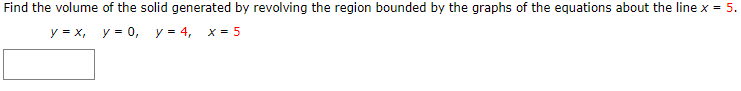# Find the volume of the solid generated by revolving the region bounded by the graphs of the equations about the line x = 5. y = x, y = 0, y = 4, x = 5

Questionhelp_outlineImage TranscriptioncloseFind the volume of the solid generated by revolving the region bounded by the graphs of the equations about the line x = 5. y = x, y = 0, y = 4, x = 5 fullscreen

### Want to see this answer and more?

Experts are waiting 24/7 to provide step-by-step solutions in as fast as 30 minutes!*

*Response times may vary by subject and question complexity. Median response time is 34 minutes for paid subscribers and may be longer for promotional offers.
Tagged in
Math
Calculus

### Applications of Integration